# Nomenclature of Alkenes

$$\newcommand{\vecs}{\overset { \rightharpoonup} {\mathbf{#1}} }$$ $$\newcommand{\vecd}{\overset{-\!-\!\rightharpoonup}{\vphantom{a}\smash {#1}}}$$$$\newcommand{\id}{\mathrm{id}}$$ $$\newcommand{\Span}{\mathrm{span}}$$ $$\newcommand{\kernel}{\mathrm{null}\,}$$ $$\newcommand{\range}{\mathrm{range}\,}$$ $$\newcommand{\RealPart}{\mathrm{Re}}$$ $$\newcommand{\ImaginaryPart}{\mathrm{Im}}$$ $$\newcommand{\Argument}{\mathrm{Arg}}$$ $$\newcommand{\norm}{\| #1 \|}$$ $$\newcommand{\inner}{\langle #1, #2 \rangle}$$ $$\newcommand{\Span}{\mathrm{span}}$$ $$\newcommand{\id}{\mathrm{id}}$$ $$\newcommand{\Span}{\mathrm{span}}$$ $$\newcommand{\kernel}{\mathrm{null}\,}$$ $$\newcommand{\range}{\mathrm{range}\,}$$ $$\newcommand{\RealPart}{\mathrm{Re}}$$ $$\newcommand{\ImaginaryPart}{\mathrm{Im}}$$ $$\newcommand{\Argument}{\mathrm{Arg}}$$ $$\newcommand{\norm}{\| #1 \|}$$ $$\newcommand{\inner}{\langle #1, #2 \rangle}$$ $$\newcommand{\Span}{\mathrm{span}}$$$$\newcommand{\AA}{\unicode[.8,0]{x212B}}$$

The molecular formula of a hydrocarbon provides information about the possible structural types it may represent. For example, consider compounds having the formula $$\ce{C5H8}$$. The formula of the five-carbon alkane pentane is $$\ce{C5H12}$$ so the difference in hydrogen content is 4. This difference suggests such compounds may have a triple bond, two double bonds, a ring plus a double bond, or two rings. Some examples are shown here, and there are at least fourteen others!Hence, as with alkanes, a consistent nomenclature system needs to be adopted that can separate the nature of these unsaturated chemicals. The simplest are the alkenes, which are hydrocarbons which have carbon-carbon double bond functional groups and are unsaturated hydrocarbons with the molecular formula is $$\ce{CnH2n}$$, which is also the same molecular formula as cycloalkanes.

##### IUPAC Rules for Alkene Nomenclature
1. The ene suffix (ending) indicates an alkene or cycloalkene.
2. The longest chain chosen for the root name must include both carbon atoms of the double bond.
3. The root chain must be numbered from the end nearest a double bond carbon atom. If the double bond is in the center of the chain, the nearest substituent rule is used to determine the end where numbering starts.
4. The smaller of the two numbers designating the carbon atoms of the double bond is used as the double bond locator.
5. If more than one double bond is present the compound is named as a diene, triene or equivalent prefix indicating the number of double bonds, and each double bond is assigned a locator number.
6. Substituent groups containing double bonds are:
• H2C=CH– Vinyl group
• H2C=CH–CH2 Allyl group

## Rule 1

Alkenes are named using the same general naming rules for alkanes, except that the suffix is now -ene. Here is a chart containing the systemic name for the first twenty straight chain alkenes.

Name Molecular formula Name Molecular formula
Ethene C2H4   Undecene C11H22
Propene C3H6   Dodecene C12H24
Butene C4H8   Tridecene C13H26

Did you notice how there is no methene? Because it is impossible for a carbon to have a double bond with nothing.

## Rule 2

The parent structure is the longest chain containing both carbon atoms of the double bond. If the alkene contains only one double bond and that double bond is terminal (the double bond is at one end of the molecule or another) then it is not necessary to place any number in front of the name.

• butane: C4H10 ($$\ce{CH3CH2CH2CH3}$$)
• butene: C4H8 ($$\ce{CH2=CHCH2CH3}$$)

If the double bond is not terminal (if it is on a carbon somewhere in the center of the chain) then the carbons should be numbered in such a way as to give the first of the two double-bonded carbons the lowest possible number, and that number should precede the "ene" suffix with a dash, as shown below.

• correct: pent-2-ene ($$\ce{CH3CH=CHCH2CH3}$$)
• incorrect: pent-3-ene ($$\ce{CH3CH2CH=CHCH3}$$)

The second one is incorrect because flipping the formula horizontally results in a lower number for the alkene.

##### Exercise $$\PageIndex{1}$$

Name the following compounds:

a: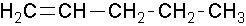b.1-pentene or pent-1-ene

2-ethyl-1-hexene or 2-ethylhex-1-ene

##### Exercise $$\PageIndex{2}$$

a: Name the following compound (hint: give the double bond the lowest possible numbers regardless of substituent placement).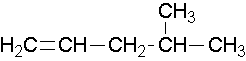b. Draw a structure for 4-methyl-2-pentene.

4-methylpent-1-ene## Rule 4

If there is more than one double bond in an alkene, all of the bonds should be numbered in the name of the molecule - even terminal double bonds. The numbers should go from lowest to highest, and be separated from one another by a comma. The IUPAC numerical prefixes are used to indicate the number of double bonds.

• octa-2,4-diene: $$\ce{CH3CH=CHCH=CHCH2CH2CH3}$$
• deca-1,5-diene: $$\ce{CH2=CHCH2CH2CH=CHCH2CH2CH2CH3}$$

Note that the numbering of "2-4" above yields a molecule with two double bonds separated by just one single bond. Double bonds in such a condition are called "conjugated", and they represent an enhanced stability of conformation, so they are energetically favored as reactants in many situations and combinations.

## Rule 4: Geometric Isomers

Double bonds can exist as geometric isomers and these isomers are designated by using either the cis / trans designation or the more flexible E / Z designation.cis Isomers have the two largest groups are on the same side of the double bond (left structure above) and trans Isomers have the two largest groups are on opposite sides of the double bond (right structure above).E/Z nomenclature

If there are 3 or 4 non-hydrogen different atoms attached to the alkene then use the E, Z system.

• E (entgegen) means the higher priority groups are opposite one another relative to the double bond.
• Z (zusammen) means the higher priority groups are on the same side relative to the double bond.

E = entgegan ("trans") Z = zusamen ("cis")

Priority of groups is based on the atomic mass of attached atoms (not the size of the group). An atom attached by a multiple bond is counted once for each bond.

fluorine atom > isopropyl group > n-hexyl group

deuterium atom > hydrogen atom

-CH2-CH=CH2 > -CH2CH2CH3

##### Example $$\PageIndex{5}$$

Try to name the following compounds using both cis-trans and E/Z conventions:

a: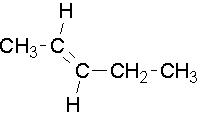b:4-methylpent-1-ene##### Example $$\PageIndex{3}$$

What is the name of this molecule?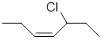###### Solution

In this diagram this is a cis conformation. It has both the substituents going upward. This molecule would be called (cis) 5-chloro-3-heptene.)

Trans would look like this

##### Example $$\PageIndex{4}$$

What is the name of this molecule?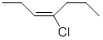###### Solution

In this example it is E-4-chloro-3-heptene. It is E because the Chlorine and the CH2CH3 are the two higher priorities and they are on opposite sides.

vi. A hydroxyl group gets precedence over the double bond. Therefore alkenes containing alchol groups are called alkenols. And the prefix becomes --enol. And this means that now the alcohol gets lowest priority over the alkene.

vii. Lastly remember that alkene substituents are called alkenyl. Suffix --enyl.

## Common names

Remove the -ane suffix and add -ylene. There are a couple of unique ones like ethenyl's common name is vinyl and 2-propenyl's common name is allyl. That you should know are...

• vinyl substituent H2C=CH-
• allyl substituent H2C=CH-CH2-
• allene molecule H2C=C=CH2
• isoprene## Endocyclic Alkenes

Endocyclic double bonds have both carbons in the ring and exocyclic double bonds have only one carbon as part of the ring.Cyclopentene is an example of an endocyclic double bond.Methylenecylopentane is an example of an exocyclic double bond.##### Exercise $$\PageIndex{1}$$

Name the following compounds:

a:b:1-methylcyclobutene. The methyl group places the double bond. It is correct to also name this compound as 1-methylcyclobut-1-ene.

1-ethenylcyclohexene, the methyl group places the double bond. It is correct to also name this compound as 1-ethenylcyclohex-1-ene. A common name would be 1-vinylcyclohexene.

##### Exercise $$\PageIndex{1}$$

Name the following compounds:

a:b:1-methylcyclobutene. The methyl group places the double bond. It is correct to also name this compound as 1-methylcyclobut-1-ene.

1-ethenylcyclohexene, the methyl group places the double bond. It is correct to also name this compound as 1-ethenylcyclohex-1-ene. A common name would be 1-vinylcyclohexene.

##### Exercise $$\PageIndex{1}$$

Draw structures for the following 2-vinyl-1,3-cyclohexadiene